# Functions (2M Questions &Solutions)|| V.S.A.Q.’S||# IPE examinations.

## Functions

QUESTION 1

Find the Domain of the following real-valued functions.

It is defined when 6x – x2 – 5 ≠ 0

⇒ x2 – 6x + 5 ≠ 0

x2 – 5x – x + 5 ≠ 0

x (x – 5) –1(x – 5) ≠ 0

(x – 5) (x – 1) ≠ 0

x ≠ 5 or x ≠ 1

∴ domain = R – {1, 5}

It is defined when 3 + x ≥ 0, 3 – x ≥ 0 and x ≠ 0

⇒ x ≥ –3, x ≤ 3 and x ≠ 0

⇒   –3≤ x, x ≤ 3 and x ≠ 0

⇒   –3≤ x ≤ 3 and x ≠ 0

x ∈ [–3, 3] – {0}

∴ domain = [–3, 3] – {0}

It is defined when x + 2 ≥ 0, 1 – x > 0 and 1 – x ≠ 0

⇒ x ≥ –2, x < 1 and x ≠ 0

x ∈ [–2, ∞) ∩ (– ∞, 1) – {0}

⇒ x ∈ [–2, 1) – {0}

∴ domain = [–2, 1) – {0}

It is defined when 4x – x2 ≥ 0

⇒ x2 – 4x ≤ 0

x (x – 4) ≤ 0

(x – 0) (x – 4) ≤ 0

x ∈ [0, 4]

∴ domain = [0, 4]

(v) f(x) = log (x2 – 4x + 3)

Given f(x) = log (x2 – 4x + 3)

It is defined when x2 – 4x + 3 > 0

⇒   x2 – 3x – x + 3 > 0

x (x – 3) –1(x – 3) > 0

(x – 3) (x – 1) > 0

x ∈ (–∞, 1) ∪ (3, ∞)

x ∈ R – [1, 3]

∴ domain = R – [1, 3]

(x + 1)(x – 1) ≥ 0 and x2 – 2x – x + 2 > 0

(x + 1) (x – 1) ≥ 0 and x (x – 2) (x – 1) > 0

x∈ (–∞, –1) ∪ (1, ∞) and x ∈ (–∞, –1) ∪ (2, ∞)

∴ domain = R – (–1, 2]

⇒ x < 0

∴ domain = (–∞, 0)

⇒ x > 0

∴ domain = (0, ∞)

QUESTION 2

If f : R→ R , g : R → R defined by f (x ) = 4x – 1, g(x) = x2 + 2 then find (i) (gof) (x) (ii) (gof) (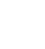)  (iii) (fof) (x)  (iv) go(fof) (0).

Sol: Given f(x) = 4x – 1, g(x) = x2 + 2

(i)  (gof) (x) = g (f (x))

= g (4x – 1)

= (4x – 1)2 + 2

= 16x2 – 8x + 1 + 2

= 16x2 – 8x + 3

= g (a + 1 – 1)

= g(a)

= a2 + 2

(iii) (fof) (x) = f (f (x))

= f (4x – 1)

= 4 (4x – 1) – 1

= 16x – 4 – 1

= 16x – 5

(iv) go(fof) (0) = g(fof) (0)

= g (f (f (0)))

= g (f (– 1))

= g (– 4 – 1)

= g (– 5)

= (– 5)2 + 2

= 25 + 2 = 27

QUESTION 3

If f and g are real valued functions defined by f(x) = 2x – 1 and g(x) = x2, then find (i) (3f – 2g) (x) (ii) (fg)(x) (iii)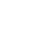(x) (iv) (f + g+ 2) (x)

Sol: Given f(x) = 2x – 1 and g(x) = x2

(i) (3f – 2g) (x) = 3f(x) – 2 g(x)

= 3(2x – 1) – 2(x2)

= 6x – 3 – 2x2

(ii) (fg)(x) = f(x) g(x)

= (2x – 1) (x2)

= 2x3 – 3x2

(iv) (f + g+ 2) (x) = f(x) + g(x) + 2

= 2x – 1 + x2 + 2

= x2 + 2x  + 1

QUESTION 4

If f = {(4, 5), (5, 6), (6, – 4)} g = {(4, – 4), (6, 5), (8,5)}, then find (i) f + g (ii) f – g (iii) 2f +4g (iv) f + 4 (v) fg (vi) f/g (vii)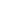(viii)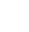(ix) f2 (x) f3

Sol: Given f = {(4, 5), (5, 6), (6, – 4)}

g = {(4, – 4), (6, 5), (8,5)}

The domain of f ∩ The Domain of g = {4, 6}

(i) (f + g) (4) = f (4) + g (4)

= 5 – 4 = 1

(f + g) (6) = f (6) + g (6)

=– 4 + 5 = 1

∴ f + g = {(4, 1), (6, 1)}

(ii) (f – g) (4) = f (4) – g (4)

= 5 – (– 4) = 5 + 4 = 9

(f – g) (6) = f (6) – g (6)

= – 4– 5   = – 9

∴ f – g   = {(4, 9), (6, – 9}

(iii) (2f +4g) (4) = 2 f (4) + 2 g (4)

= 2(5) + 4 (– 4)

= 10 – 16

=– 6

(2f +4g) (6) = 2 f (6) + 2 g (6)

= 2(– 4) + 4 (5)

= – 8 + 20

=12

∴ (2f +4g) = {(4, – 6), (6, 12)}

(iv) (f + 4) (4) = f (4) + 4 = 5 + 4 = 9

(f + 4) (5) = f (5) + 4 = 6 + 4 = 10

(f + 4) (6) = f (6) + 4 = – 4 + 4 = 0

∴ (f + 4) = {(4, 9), (5, 10), (6, 0)}

(v) fg (4) = f (4) g (4) = (5) (– 4) =– 20

fg (6) = f (6) g (6) = (– 4) (5) =– 20

∴ fg = {(4, – 20), (6, – 20)}

(vi) f/g (4) = f(4)/g(4) = 5/ – 4 = – 5/4

f/g (6) = f(6)/g(6) = – 4/ 5

∴ f/g = {(4, – 5/4), (6, – 4/ 5)}(ix) f2(4) = (f (4))2 = (5)2 = 25

f2(5) = (f (5))2 = (6)2 = 36

f2(6) = (f (6))2 = (– 4)2 = 16

∴ f2 = {(4, 25), (5, 36), (6, 16)}

(x) f3(4) = (f (4))3 = (5)3 = 125

f3(5) = (f (5))3 = (6)3 = 216

f3(6) = (f (6))3 = (– 4)3 = –64

∴ f3 = {(4, 125), (5, 216), (6, –64)}

QUESTION 5

If A = {0, π/6, π/4, π/3, π/2} and f: A→ B is a surjection defined by f(x) = cos x, then find B.

Sol: Given A = {0, π/6, π/4, π/3, π/2}

f(x) = cos x

f (0) = cos (0) = 1

f(π/3) = cos (π/3) = 1/2

f(π/2) = cos (π/2) = 0

QUESTION 6

If A = {–2, –1, 0, 1, 2} and f: A→ B is a surjection defined by f(x) =x2 + x + 1, then find B.

Sol: Given A = {–2, –1, 0, 1, 2} and f(x) = x2 + x + 1

f (–2) = (–2)2 + (–2) + 1= 4 – 2 + 1 = 3

f (–1) = (–1)2 + (–1) + 1= 1 – 1 + 1 = 1

f (0) = (0)2 + (0) + 1= 0 + 0 + 1 = 1

f (1) = (1)2 + (1) + 1= 1 + 1 + 1 = 3

f (2) = (2)2 + (2) + 1= 4 + 2 + 1 = 7

∴ B = {1, 3, 7}

QUESTION 7

If A = {1, 2, 3, 4} and f: A→ B is a surjection defined by f(x) =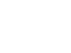then find B.

QUESTION 8

If f(x) = 2, g(x) = x2, h(x) = 2x ∀ x ∈ R, then find (fo(goh)) (x)

Sol: Given f(x) = 2, g(x) = x2, h(x) = 2x

(fo(goh)) (x) = fo (g (h (x))

= f (g (h (x))

= f(g(2x)

= f((2x)2)

= f(4x2) = 2

QUESTION 9

If f: R→ R, g: R → R defined by f (x) = 3x – 2, g(x) = x2 +1 then find (i) (gof-1) (2) (ii) (gof) (x – 1)

Sol: Given f: R→ R, g: R → R defined by f (x) = 3x – 2, g(x) = x2 +1

(i) Let y = f(x) ⟹ x = f-1(y)

y = 3x – 2

y + 2 = 3x

x = (y + 2)/3

f-1(y) = (y + 2)/3

∴ f-1(x) = (x + 2)/3

Now

(gof-1) (2) = g(f-1(2))

= g ((2 + 2)/3)

= g (4/3)

= (4/3)2 + 1 = 16/9 + 1 = 25/9

(ii) (gof) (x – 1) = g (f (x – 1))

= g [ 3(x – 1) – 2)]

= g (3x – 3 – 2)

=g (3x – 5)

= (3x – 5)2 + 1

= 9x2 – 30x + 25 + 1

= 9x2 – 30x + 26

QUESTION 10

If f: N→ N defined by f (x) = 2x + 5, Is onto? Explain with reason.

Sol: Given f (x) = 2x + 5

Let y = f(x) ⟹ x = f-1(y)

y = 2x + 5

2x = y – 5

x = (y – 5)/2 ∉ N

∴ f(x) is not onto

QUESTION 11

Find the inverse of the following functions

(i) If a, b ∈ R, f: R→ R defined by f(x) = ax + b

Given function is f(x) = ax + b

Let y = f(x) ⟹ x = f-1(y)

y = ax + b

y – b = ax

x = (y – b)/a

f-1(y) = (y – b)/a

∴ f-1(x) = (x – b)/a

(ii) f: R→ (0, ∞) defined by f(x) = 5x

Given function is f(x) = 5x

Let y = f(x) ⟹ x = f-1(y)

y = 5x

Let y = f(x) ⟹ x = f-1(y)

x = 2y

f-1(y) = 2y

∴ f-1(x) = 2x

(iv) f: R→ R defined by f(x) = e4x + 7

Given function is f(x) = e4x + 7

Let y = f(x) ⟹ x = f-1(y)

y = e4x + 7

(v) f: R→ R defined by f(x) = (2x + 1)/3

Given function is f(x) = (2x + 1)/3

Let y = f(x) ⟹ x = f-1(y)

y= (2x + 1)/3

3y = 2x + 1

2x = 3y – 1

x = (3y – 1)/2

f-1(y) = (3y – 1)/2

∴ f-1(x) = (3x – 1)/2

QUESTION 12

If f: R→ R defined by f(x) =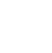, then show that f (tan θ) = cos 2θ

∴  f (tan θ) = cos 2θ

QUESTION 13

QUESTION 14

If the function f: R→ R defined by f(x) =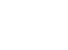, then show that f (x + y) + f (x – y) = 2 f(x) f(y).

∴ f (x + y) + f (x – y) = 2 f(x) f(y)

QUESTION 15

Sol: Given function is f(x) = cos (log x)= cos (log x) cos (log y) – cos (log x) cos (log y)

= 0

Hence proved

QUESTION 16

Find the range of the following real-valued functions

Sol:

ey > 0

∴ Range of f(x) is R

It is defined when x – 2 ≠ 0

⟹ x ≠ 2

Domain = R – {2}

y = x + 2

if x = 2 ⟹ y = 4

∴ Range of f(x) is R – {4}

QUESTION 17

Find the domain and range of the following real valued functions

Sol:

It is defined when 1 + x2 ≠ 0

⟹ x2 ≠ – 1

x ∈ R

∴ domain of f(x) is R

y (1 + x2) = x

y + x2y = x

x2 y – x + y = 0

It is defined when 1 – 4y2 ≥ 0 and 2y ≠ 0

⟹ 4y2 – 1 ≤ 0 and y ≠ 0

(2y – 1) (2y + 1) ≤ 0 and y ≠ 0

(y – 1/2) (y + 1/2) ≤ 0 and y ≠ 0

–  ½≤ y ≤ ½ and y ≠ 0

∴ Range of f(x) is [– ½, ½] – {0}

It is defined when 9 – x2 ≥ 0

⟹ x2 – 9 ≤ 0

(x + 3) (x – 3) ≤ 0

–  3 ≤ y ≤ 3

∴ the domain of f (x) is [– 3, 3]

y2 = 9 – x2

x2 = 9 – y2

It is defined when 9 – y2 ≥ 0

⟹ y2 – 9 ≤ 0

(y + 3) (y – 3) ≤ 0

–  3 ≤ y ≤ 3

y ∈ [– 3, 3]

∴ Range of f (x) is [0, 3] (∵ y ≥ 0)

clearly, x ∈ R

∴ domain of f(x) is R

If x = 0, then y = 1

If x = – 1, then y = 1

If x = 1, then y = 3

If x = – 2, then y = 3

If x = 2, then y = 5

∴ Range of f (x) is [1, ∞)

(iv) f(x) = [x]

clearly Domain = R  and Range = Z

QUESTION 18.

Define (i) One – One function (ii) Onto function (iii) Bijection (iv) Even and Odd functions

(i) One – One Function: one – one, if every element of A has a unique image in B.

(ii) Onto function: A function f: A→ B is said to be onto if ∀ y ∈ B there exists x ∈ A such that f(x) = y.

(iii) Bijection: A function f: A→ B is said to be Bijection if it is both one-one and onto.

(iv) Even and Odd functions:

If f(–x) = f(x), then f(x) is even function

If f(– x)

= – f(x), then f(x) is odd function